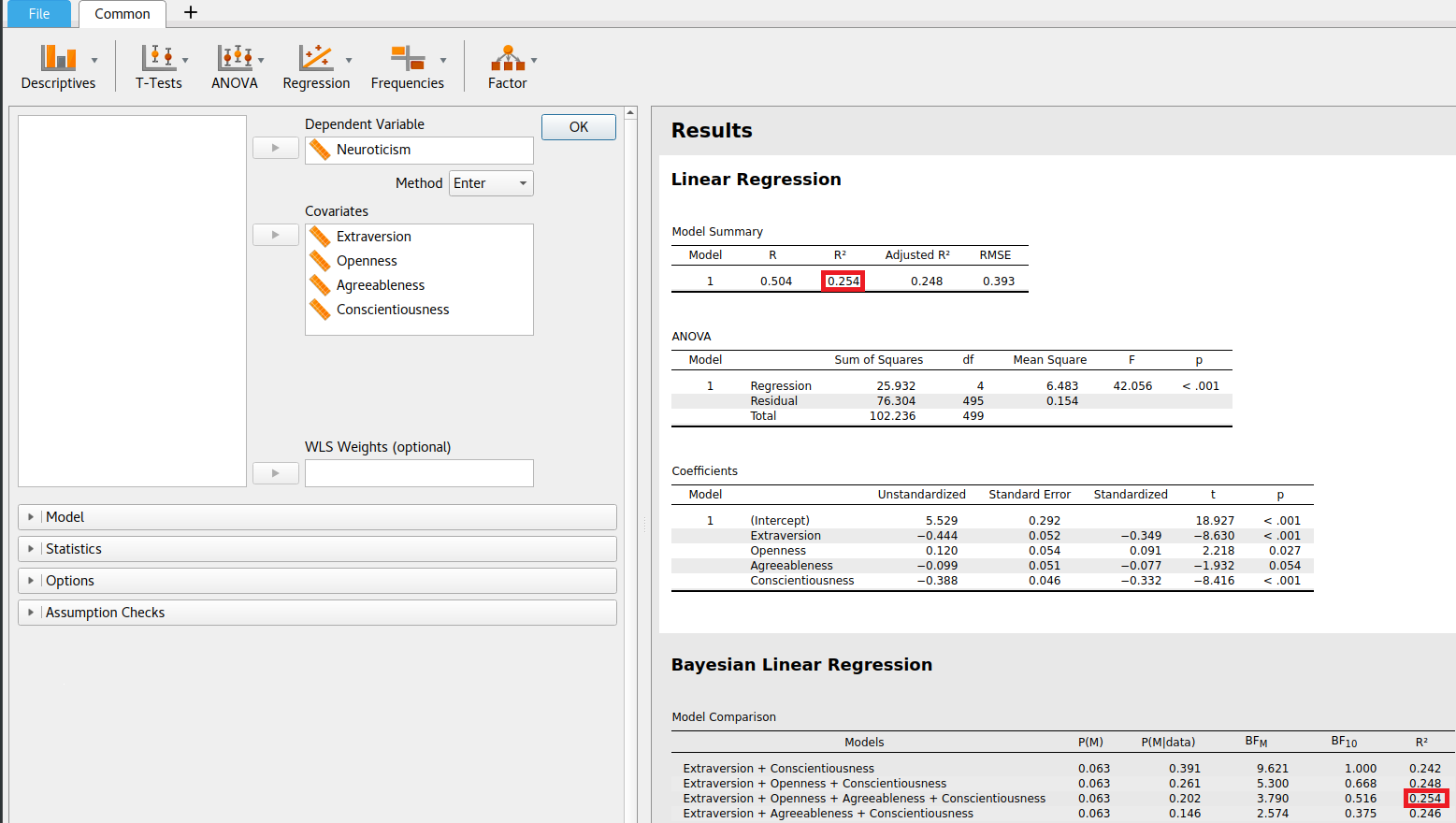#### Howdy, Stranger!

It looks like you're new here. If you want to get involved, click one of these buttons!

Supported by

# R-squared for Bayesian linear regression in JASP

Hello,
Would it be possible to know how the R-squared for Bayesian linear regression is calculated in JASP?
Can I use the R-squared for a Bayesian linear regression to compute an effect size or should I stick to the traditional R-squared?
Thank you very much,

Best,
Sophie

• not for, but from*

• Hi Sophie,

R-squared is calculated in the usual way and the results for any particular model are identical to the frequentist one (as illustrated by the screenshot).The posterior means of the parameters are used in the calculation of the R-squared. While technically, R-squared should be represented with a posterior distribution, we only report the posterior mean.
The R-squared of a particular model can be used to computed an effect size, albeit that any model uncertainty is ignored. It is possible to compute a model averaged effect size, but this is not done by JASP at the moment.

The source code for the calculation of the R-squared can be found here (function Fitmodel).

Best,
Don

Thanked by 1EJ